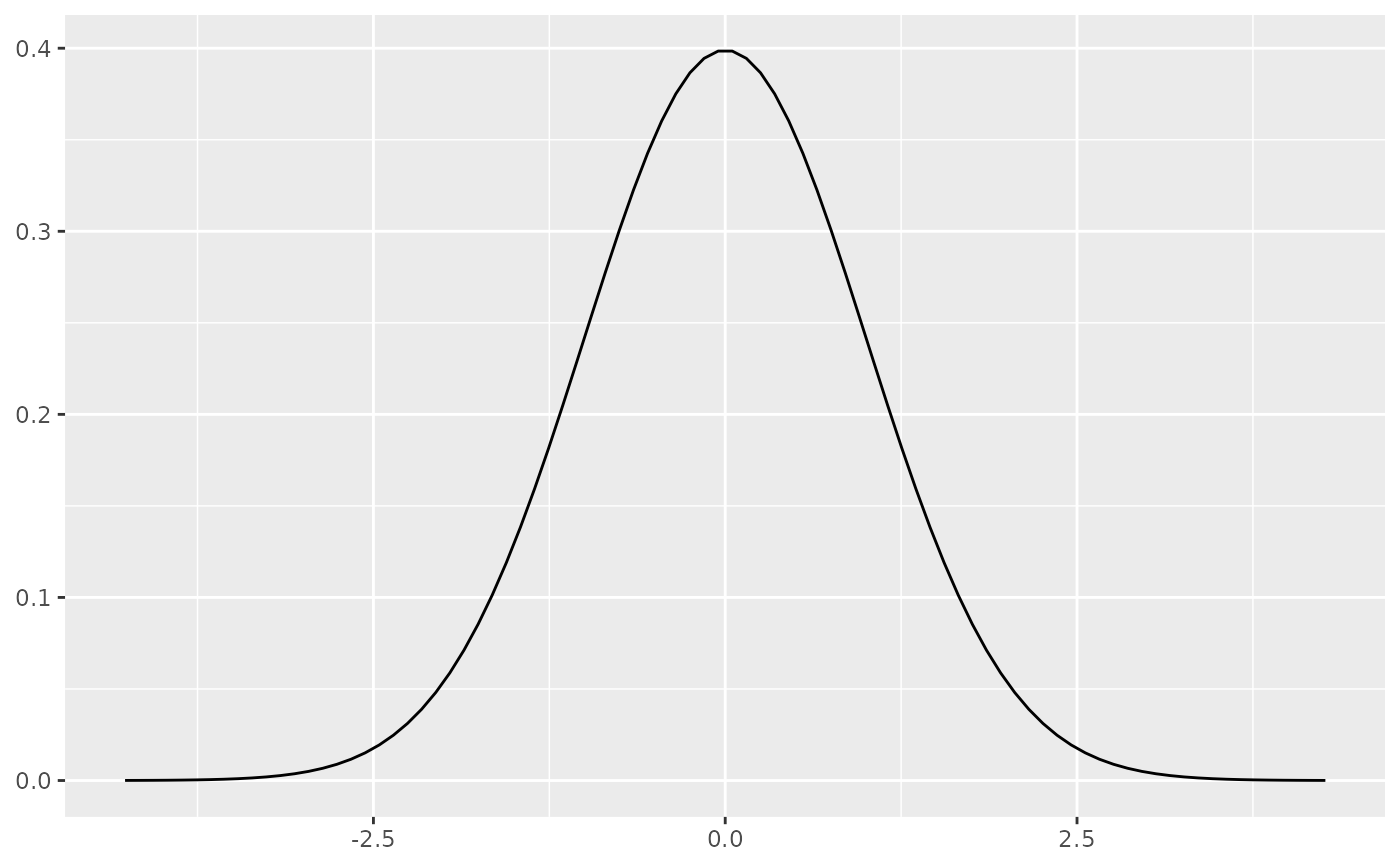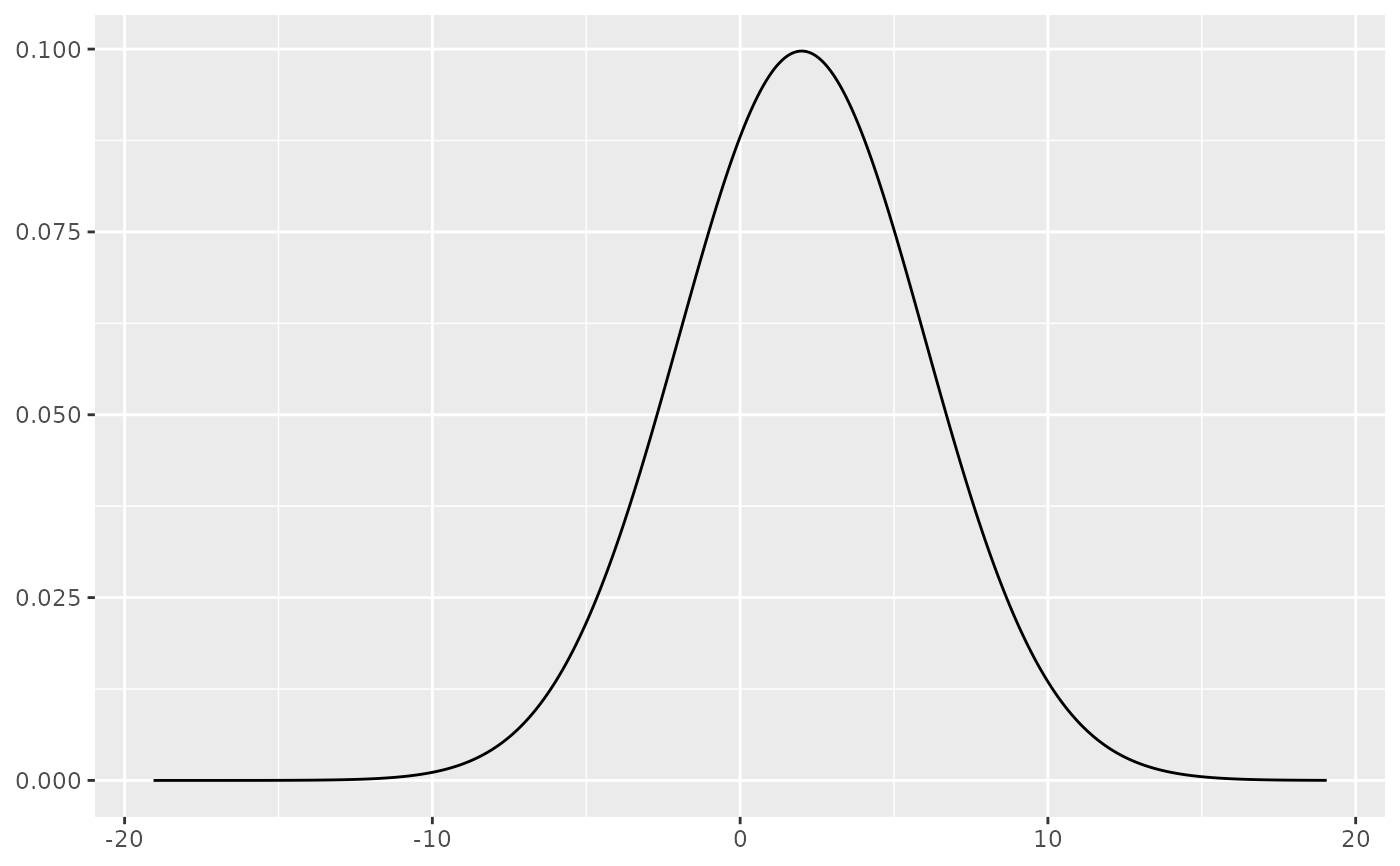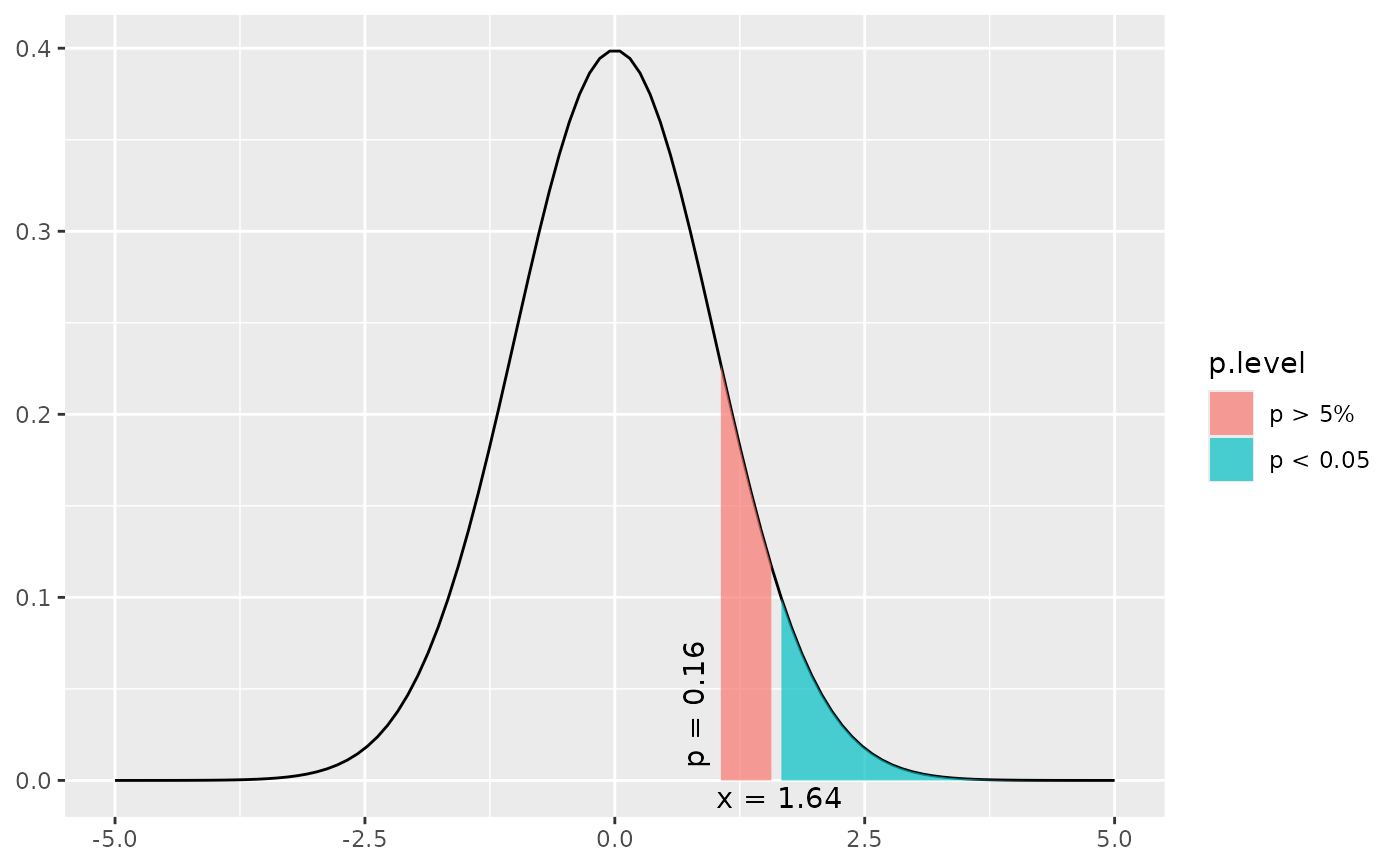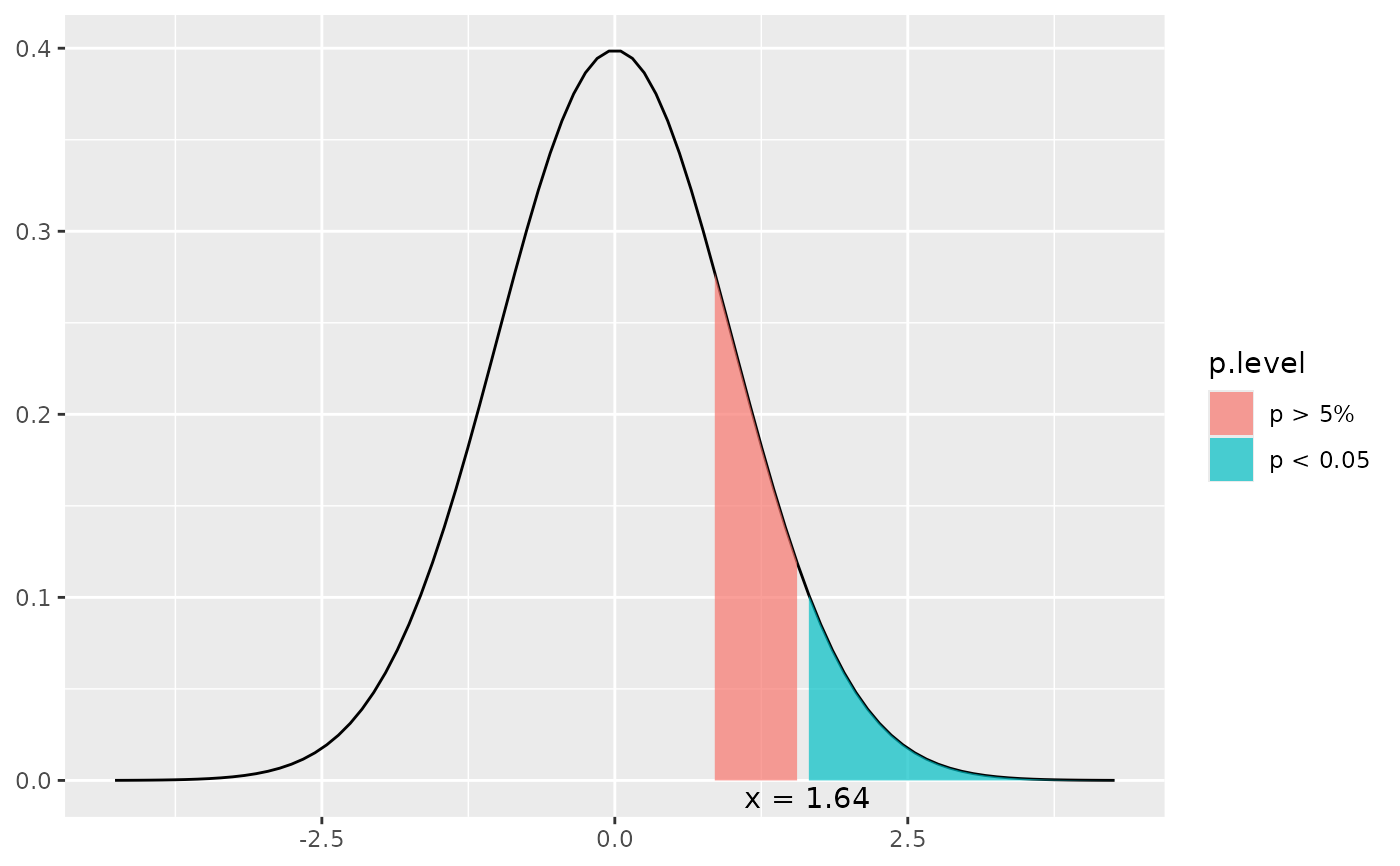This function plots a simple normal distribution or a normal distribution with shaded areas that indicate at which value a significant p-level is reached.

dist_norm(
norm = NULL,
mean = 0,
sd = 1,
p = NULL,
xmax = NULL,
geom.colors = NULL,
geom.alpha = 0.7
)

Arguments

norm

Numeric, optional. If specified, a normal distribution with mean and sd is plotted and a shaded area at norm value position is plotted that indicates whether or not the specified value is significant or not. If both norm and p are not specified, a distribution without shaded area is plotted.

mean

Numeric. Mean value for normal distribution. By default 0.

sd

Numeric. Standard deviation for normal distribution. By default 1.

p

Numeric, optional. If specified, a normal distribution with mean and sd is plotted and a shaded area at the position where the specified p-level starts is plotted. If both norm and p are not specified, a distribution without shaded area is plotted.

xmax

Numeric, optional. Specifies the maximum x-axis-value. If not specified, the x-axis ranges to a value where a p-level of 0.00001 is reached.

geom.colors

user defined color for geoms. See 'Details' in plot_grpfrq.

geom.alpha

Specifies the alpha-level of the shaded area. Default is 0.7, range between 0 to 1.

Examples

# a simple normal distribution
dist_norm()# a simple normal distribution with different mean and sd.
# note that curve looks similar to above plot, but axis range
# has changed.
dist_norm(mean = 2, sd = 4)# a simple normal distribution
dist_norm(norm = 1)# a simple normal distribution
dist_norm(p = 0.2)